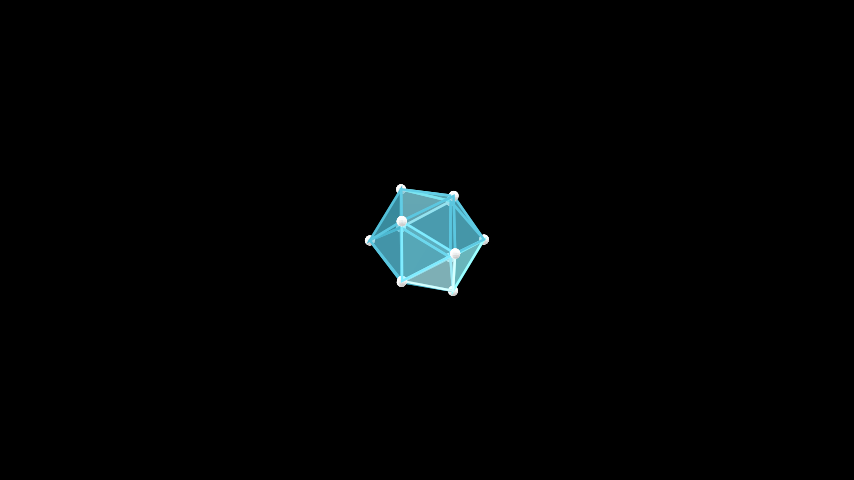# Icosahedron#

Qualified name: `manim.mobject.three\_d.polyhedra.Icosahedron`

class Icosahedron(edge_length=1, **kwargs)[source]#

Bases: `Polyhedron`

An icosahedron, one of the five platonic solids. It has 20 faces, 30 edges and 12 vertices.

Parameters

edge_length (float) – The length of an edge between any two vertices.

Examples

Example: IcosahedronScene```from manim import *

class IcosahedronScene(ThreeDScene):
def construct(self):
self.set_camera_orientation(phi=75 * DEGREES, theta=30 * DEGREES)
obj = Icosahedron()
 `animate` Used to animate the application of any method of `self`. `animation_overrides` `color` `depth` The depth of the mobject. `fill_color` If there are multiple colors (for gradient) this returns the first one `height` The height of the mobject. `n_points_per_curve` `sheen_factor` `stroke_color` `width` The width of the mobject.